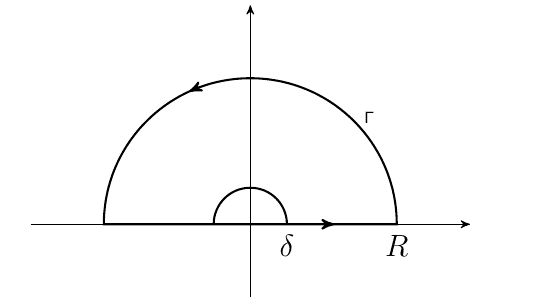# Proving integral on small contour is equal to 0.

Consider the integral:

$$\int_{0}^{\infty} \frac{\log^2(x)}{x^2 + 1} dx$$$R$ is the big radius, $\delta$ is the small radius.

Actually, lets consider $u$ the small radius. Let $\delta = u$

Ultimately the goal is to let $u \to 0$

We can parametrize,

$$z = ue^{i\theta}$$

$$\int_{\delta} f(z)dz = (-)\cdot\int_{0}^{\pi} \frac{(i\theta + \log(u))^2\cdot (uie^{i\theta})}{(ue^{i\theta})^2 + 1} d\theta$$

$$\left | \int_{0}^{\pi} \frac{(i\theta + \log(u))^2\cdot (uie^{i\theta})}{(ue^{i\theta})^2 + 1} d\theta \right | \le \int_{0}^{\pi} \frac{|(i\theta + \log(u))|^2\cdot(u)}{|(ue^{i\theta})^2 + 1 |} d\theta$$

$$|(ue^{i\theta})^2 + 1 | < u^2 + 1$$

$$\frac{1}{u^2 + 1} < \frac{1}{|(ue^{i\theta})^2 + 1 |}$$

Since the maximum value of $\theta$ is $\theta = \pi$

$$|(i\theta + \log(u))| = \sqrt{\log^2(u) - \theta^2} \le \sqrt{\log^2(u) + \pi^2}$$

So:

$$|(i\theta + \log(u))|^2 \le \log^2(u) + \pi^2$$

Then:

$$|(i\theta + \log(u))|^2 \le \log^2(u) + \pi^2$$

For values $u$ near $0$.

$$(u)|(i\theta + \log(u))|^2 \le (\log^2(u) + \pi^2)u \le (\pi^2)u + 5\pi^2$$

Therefore,

$$\frac{|\log(z)|}{|z^2 + 1|} \le \frac{(\pi^2)u + 5\pi^2}{u^2 + 1}$$

Then we take the limit as $u \to 0$ which makes the RHS of the inequality 0.

hence the LHS upperbound is $0$.

So is the contour integral around the small semi circle $\delta$ = 0?

How do I do this?

Thanks